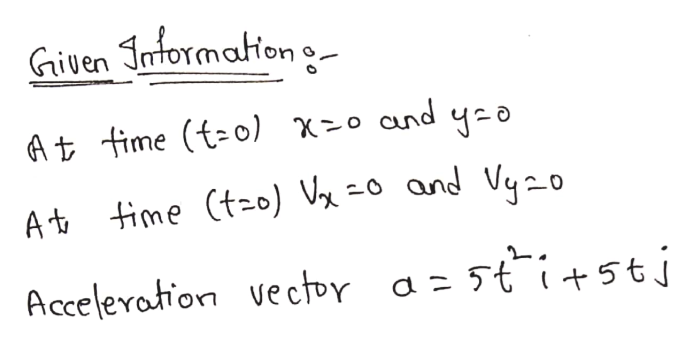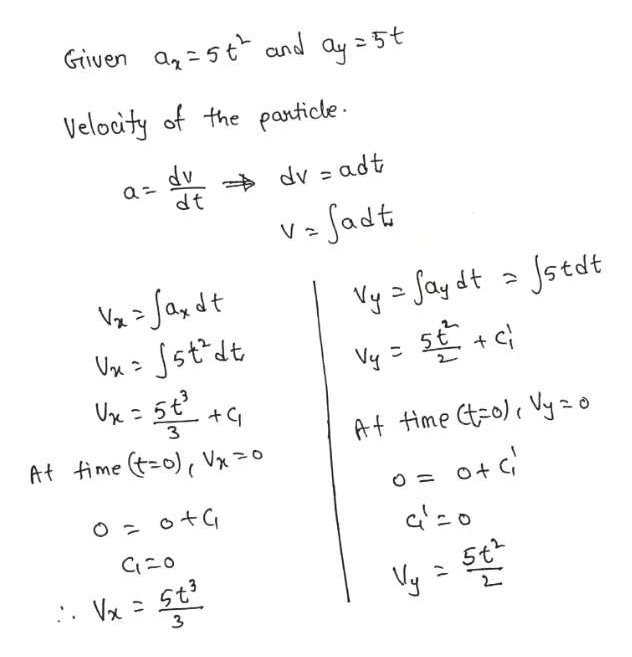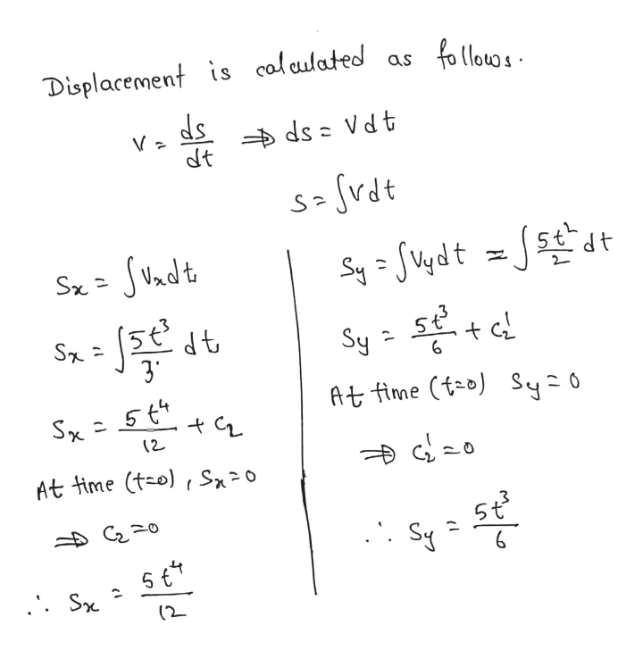A particle is at rest and at x=0 and y=0 at time = 0. The particle then moves in the x-y plane with an acceleration in m/s^2 of a = 5t^2 i + 5t j , where t is in seconds. Determine the magnitude of the particle's velocity vector in m/s at instant the y-component of the position vector ry = 8 meters.

Question

A particle is at rest and at x=0 and y=0 at time = 0. The particle then moves in the x-y plane with an acceleration in m/s^2 of a = 5t^2 i + 5t j , where t is in seconds. Determine the magnitude of the particle's velocity vector in m/s at instant the y-component of the position vector ry = 8 meters.

Step 1help_outlineImage TranscriptioncloseGiven Jntormation - At fime (tzo) x-0 cand yo At time tzo) Vx =o and Uyzo Acceleration vectpr a z 5ti+5tj fullscreen
Step 2help_outlineImage TranscriptioncloseGiven a5t and ay 5t Velodty of the panticle a dv dt dv adt V -Ja,dt VxSstdt Jstdt Vyay dt 5 Vy At time Cto) Vyzo At time to), Vxo C20 . V St fullscreen
Step 3help_outlineImage TranscriptioncloseDisplacement is calculated as ds dt ds Vd t S vdt SVndt Sx= S- 37 Sy 6 At fime (to) Sy 0 (2 At time (t-o) S0 5t S C20 (2 fullscreen

Want to see the full answer?

See Solution

Want to see this answer and more?

Our solutions are written by experts, many with advanced degrees, and available 24/7

See Solution
Tagged in

Kinematics